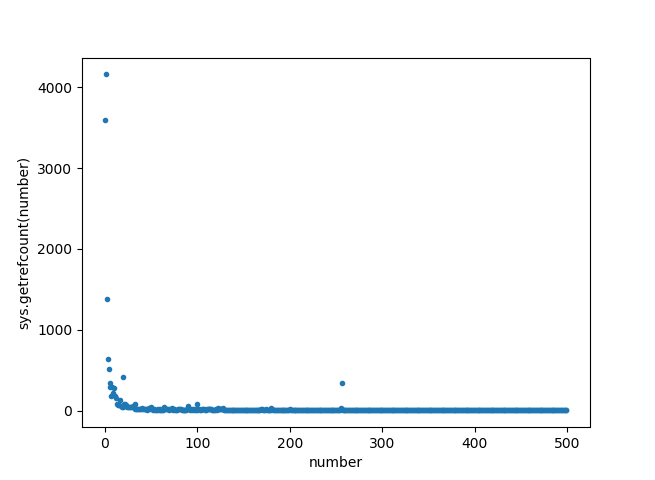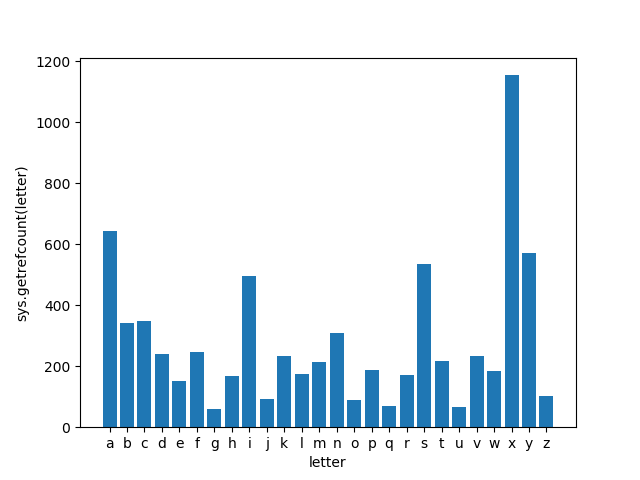# Mystery of Python getrefcount() | Reference Count & Memory Management

Have you ever wondered- How the memory is managed in Python?

Or what is reference count in Python?

Without wasting any further time, let’s start point by point…## What is Python Reference Count?

For the sake of simplicity, the reference count is nothing but the number of times Python object is used.

How is reference count calculated?

The Python `getrefcount()` is the function present inbuilt with the Python module `sys`. This functions takes Python object as an input and returns the number of references present for the given Python object.

Here, input to the `getrefcount()` can be a variable name, value, function, class and anything else comes under Python object.

Let’s take an example…

```import sys
print(sys.getrefcount(1556778))```

Output:

3

This means the integer value ‘1556778’ is used 3 times.

You might be curious… how does it comes 3 times, even if you have used the value only once?

### How is the reference count calculated?

The reference count is calculated based on the two factors…

• Number of times object used in the bytecode
• If the same object used earlier, number of object reference from earlier code  (can be in the same program or in a background process of Python)

Let’s bend into some technical detail…

1. Reference Count from Bytecode:

When you run any Python program, it gets interpreted into the bytecode. The reference count of the object is calculated based on the number of times object is used in the bytecode (not from your high-level program code).

You can also check the bytecode of your program using the dis module. It disassembles the Python bytecode.

Below is code to get the bytecode of the Python program.

```import dis
import sys

print(compile("sys.getrefcount(1556778)", '', 'single').co_consts)
print(dis.dis(compile("sys.getrefcount(1556778)", '', 'single')))
print(sys.getrefcount(1556778))```

Output:

```(1556778, None)
9 CALL_FUNCTION 1
12 PRINT_EXPR
16 RETURN_VALUE
None
3```

Here, `single` is a mode of Python interpreter.

There are 3 references here- one from the `co_consts` tuple on the code object, one on the stack (from the `LOAD_CONST` instruction), and one for the `sys.getrefcount()` method itself.

1. Reference Count from other parts of the Code:

If the same object is used in the other part of the code, it will be counted in the reference count of the given object.

Even, there are multiple cumbersome operations goes running in background Python. It may possible that this object is used in the background of your running program. It is also counted as a reference to the object.

The output (reference count) may vary from system to system.

### Reference Count for Variable and Function:

When you pass the variable as a parameter to the function, reference count for the variable object is incremented. When the control goes out of the function, the reference count is decremented.

```import sys
a =10
print(sys.getrefcount(a)) #17

def func(b):
print(sys.getrefcount(a)) #19

func(a)
print(sys.getrefcount(a)) #17```

Note: Reference count is shown as 19 instead of 18 because of variable ‘a’ is used two times in function- as a parameter to the function `func()`; as a parameter to the function `sys.getrefcount()`.

### Reference Count for Python List

Along with the list object, every element in the list has a separate reference count.

When you delete a list or if the lifetime of the list expires, the reference count of each element in the list goes down by one.

```import sys

liAbc = ['a', 'b', 'c']

print(sys.getrefcount('a')) #14
print(sys.getrefcount('b')) #12
print(sys.getrefcount('c')) #23

del liAbc

print(sys.getrefcount('a')) #13
print(sys.getrefcount('b')) #11
print(sys.getrefcount('c')) #22```

More detail about the list, you can read Python list vs tuple.

### When does the reference count increase?

• while assigning operator
• while passing the value as an argument to the function
• appending object to the list

## Use of the reference count for Memory Management in Python:

Python uses dynamic memory allocation. While declaring a variable object, you don’t need explicitly allocate the memory. When the object is no more used in the program, the variable is deleted.

There are two questions arises…

• While creating the object, what if the object already exists in memory?
• While deleting the object, how the system will know if the object is no more used?

And, here comes the use of reference count.

How does Python count references use for Memory Management?

Python count the reference for each object. When you use that object again, the reference count is incremented.

When the reference object comes out of scope, the reference count is decremented.

When the reference count reaches zero, means the Python object is not in use. The memory which is assigned to the object gets deleted.

### Reference Count for Integer (Immutable Object)

Integer is one of the numeric data types in Python.

When you create an integer object, the value of the object is saved in memory to use in the program. The reference count is set.

When you assign the same integer value to another variable, the reference count increases.

It also saves the computing resources by using a single place to store the value and assigned to all the variable storing the same value in the program.

And we know that integer is immutable datatype in Python. So we can not change the value of the integer. The new value is stored in different memory with the new reference count.

```import sys
print(sys.getrefcount(55)) #4

var = 55
print sys.getrefcount(55) #5

var = var + 1
print sys.getrefcount(55) #4```

In the above program, the value of the variable var is incremented (you can change it to any other value or delete the variable). As Integer is immutable, we can not update the integer value, instead, it stores at a different place and decrements the reference count of previous value by one.

Now, what if you use smaller integer value?

```import sys
print(sys.getrefcount(1)) #97
print(sys.getrefcount(2)) #76
print(sys.getrefcount(3)) #30```

This, means integer value 1 is used 97 times, 2 is used 76 and 3 is used 30 times.

There are multiple cumbersome operations goes running on Python background. So these values are used. The output may vary from system to system.

## Lets’ play the getrefcount() with different inputs:

To find the pattern for a number of times Python object is used, we can plot the graph for a range of input objects.

1. Write a program to plot a graph based on reference count for integer values (say 1 to 500) used in the Python.
```import sys
import matplotlib.pyplot as plt

#calculate the values for x and y axis
x = range(500)
y = [sys.getrefcount(i) for i in x]

fig, ax = plt.subplots()
plt.plot(x, y, '.')

#set lable for x axis
ax.set_xlabel("number")
#set lable for y axis
ax.set_ylabel("sys.getrefcount(number)")

#plot the graph
plt.show()```From the graphs, it is clear that there are more numbers of reference count for smaller numbers. A couple of initial smaller values have a reference count more than 3000. Means, smaller numbers are used widely running Python in the background.

1. Similarly, let’s plot the reference counts graph for 26 English letters.
```import sys
import matplotlib.pyplot as plt

#string with all character letters
strLet = "abcdefghijklmnopqrstuvwxyz"
refs = [sys.getrefcount(l) for l in strLet]
y_pos = range(len(strLet))
plt.bar(y_pos, refs, align='center')
plt.xticks(y_pos, letters)

#set lable for x axis
plt.xlabel("letter")
#set lable for y axis
plt.ylabel('sys.getrefcount(strLet)')

#plot the graph
plt.show()```We are more obsessed with the letter ‘x’ and it is used for many variable declarations. If you look at the graph it holds true. The ‘x’ as the object is used more than 1100 in Python.

As Python is case sensitive language, you will get the difference reference count (so the different graph) for small and big caps letters.

1. Take some popular keyword in Python, count and plot the reference count.

How can we exclude “Python” itself?

```import sys
for w in ["python", "version", "error", "var", "reference"]:
print w, sys.getrefcount(w)```

```('python', 6)
('version', 12)
('error', 47)
('var', 9)
('reference', 6)```

You can try some other keywords as well.

### Keys Points to remember about Python Reference Count:

• You may get a difference reference count for the same object on the different Python system. It solely depends on the number of times object is used on your system.
• If you declare the object as global (declared outside of any block, class or functions), can never have reference count zero.
• Value of the reference count is always one higher than the one you expect as it also counts reference for an object passing to function `sys.getrefcount()` itself.
• For Python memory management, the reference count is used. When reference count of the any Python object goes down to zero, the memory assigned to the object is deleted.
• You can relate the Reference with the pointer concepts in C programming.

That’s all!

The understanding reference count is very for memory management. If you find this article fruitful, kindly share with your friends.

I have tried to address answers to multiple daunting questions about Python `getrefcount()` function, reference count and memory management. If you have any doubt, feel free to write in the comment section.